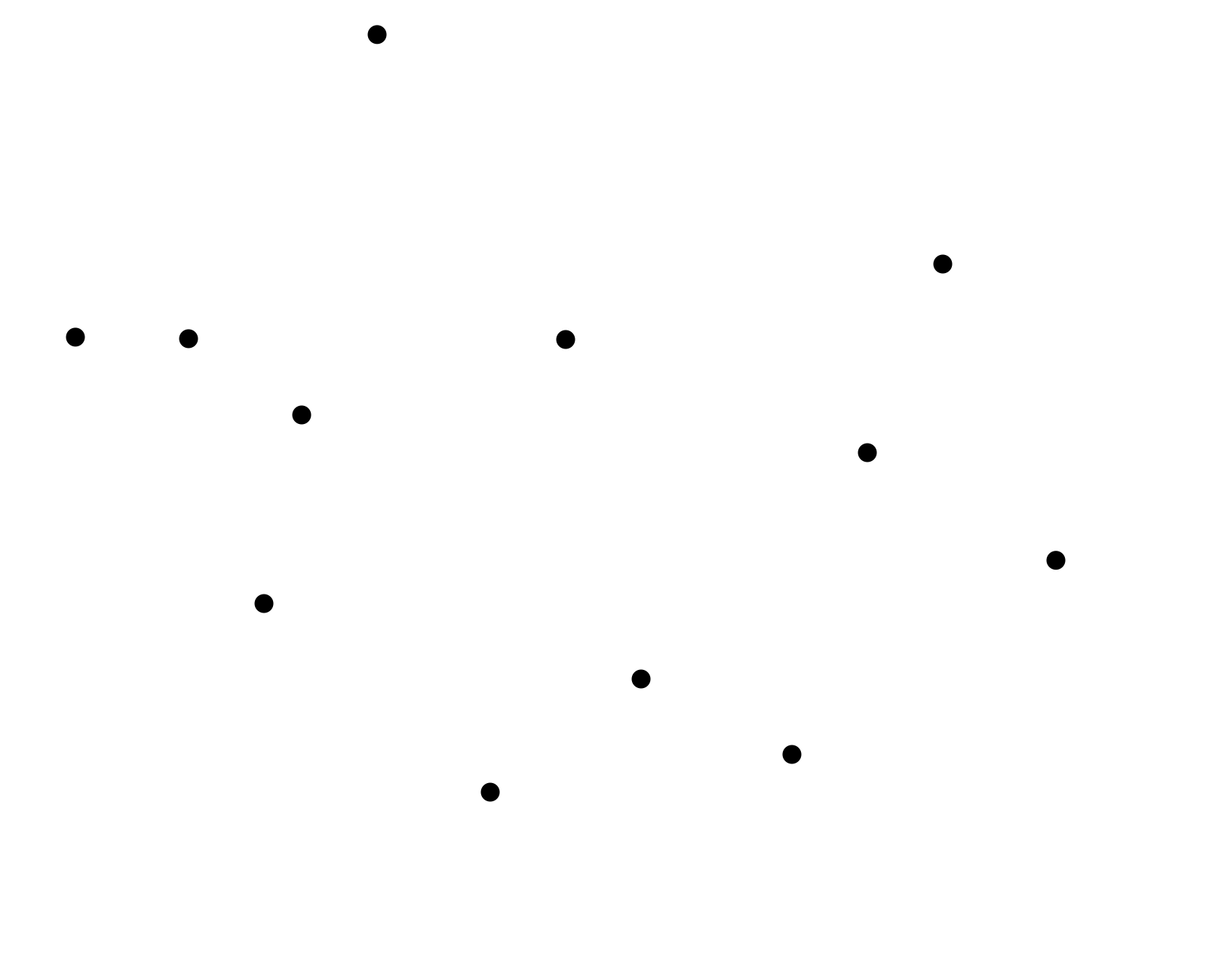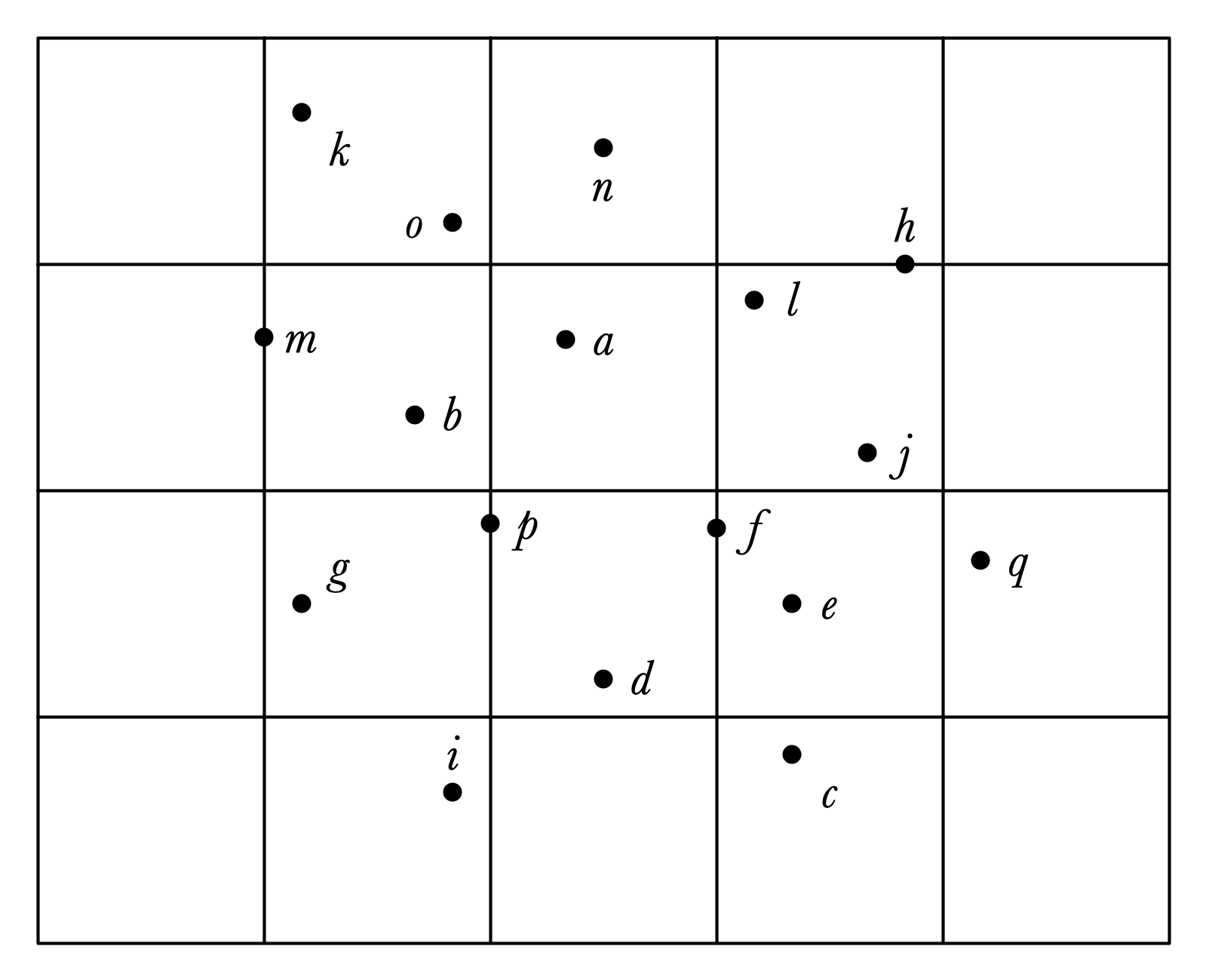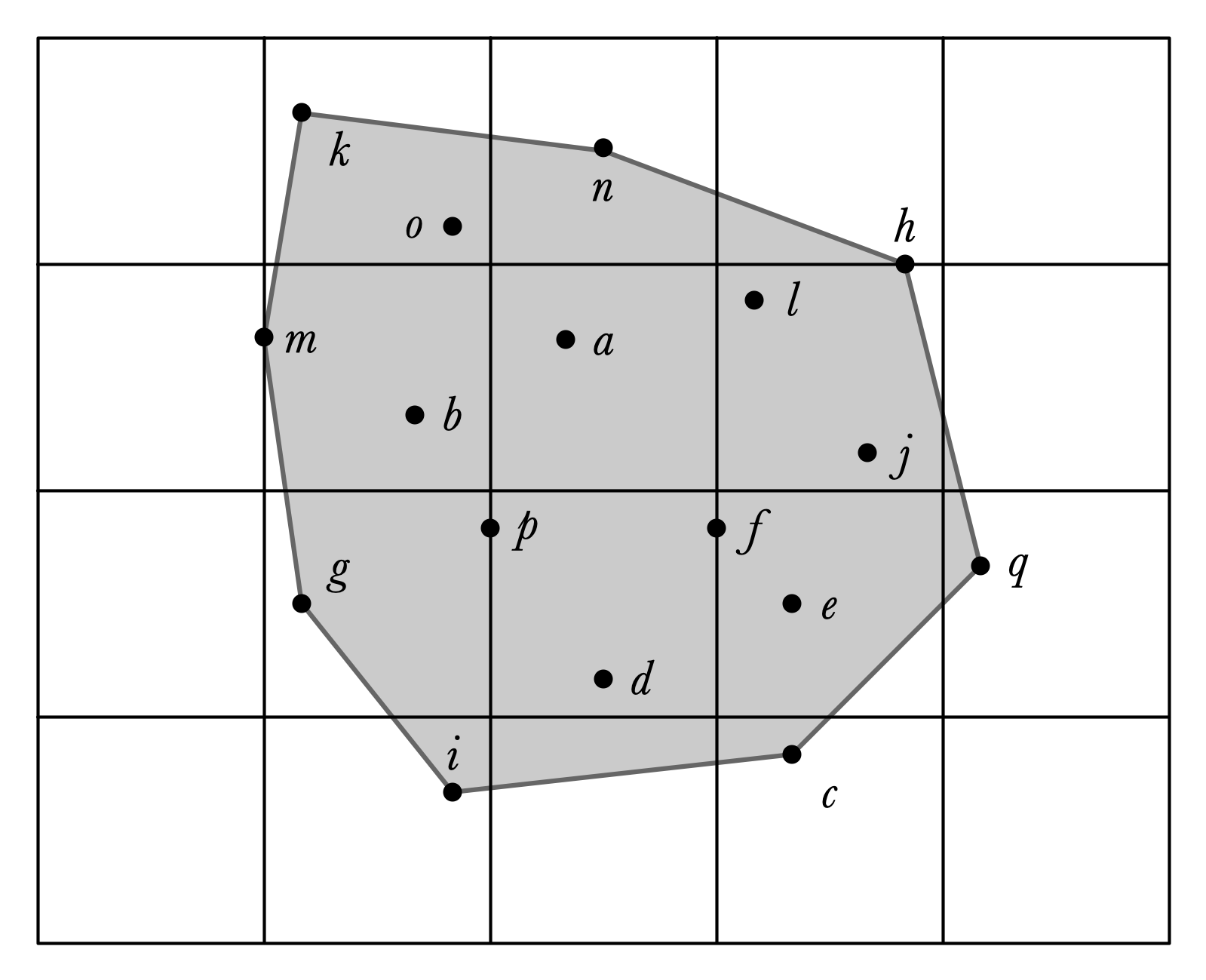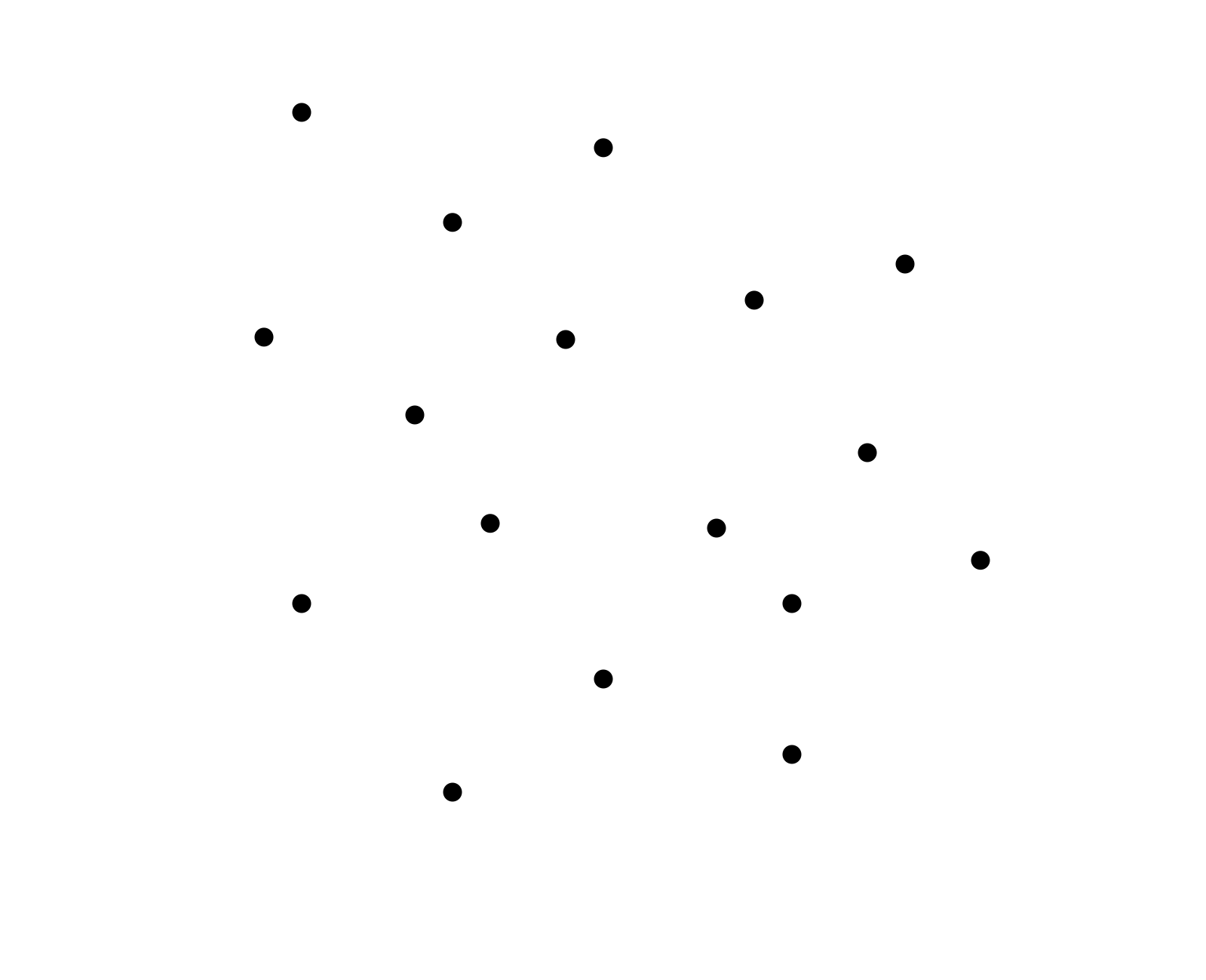# Lecture 09: Convex Hulls

## Annoucements

1. Office hours canceled today
2. Re-submission for assignments 1 and 2 open Wednesday to Friday
3. Assignment 06 can be done in pairs!
• partner questionnaire today by Wednesday
• Assignment 06 due 03/24

## Outline

1. Convex Hulls
2. Activity: Finding the Convex Hull
3. Graham’s Scan Algorithm

## Last Time

Depth-first Search: A Case Study in Visualizing Algorithms

• Building a graph interactively
• Stepping through an execution
• Visualizing each step

## Today

Convex hulls:

• fundamental problem in computational geometry
• topic of Assignment 06

## Convex Hulls, Two Views

Given a finite set of points in the plane:

• What is the smallest convex region that contains all of the points?
• What is the minimum perimeter of a polygon that contains the points?

## Visualizing Convex Hulls## Which Points are on the Boundary?## Convex Hull Problem

Input:

• set of points in plane
• $(x, y)$-coordinates of each point

Output:

• a sequence of points $(x_1, y_1), (x_2, y_2), \ldots, (x_k, y_k)$ that define the “boundary” of the set of points
• path around $(x_1, y_1), (x_2, y_2), \ldots, (x_k, y_k)$ surrounds all points in the set in clockwise order
• the bounded region is convex

## Convex Hull Output Depiction## Activity!

Find the Convex Hull!

## Graphed Points## With the Convex Hull## Observe

It is easy to find by hand once you’ve graphed the points.

Question. How to program a computer to find the convex hull?

## Notation

• $X = {(x_1, y_1), (x_2, y_2), \ldots, (x_n, y_n)}$ a set of points
• $CH(X)$ is the convex hull of $X$
• more specifically, sequence of points in $X$ that are vertices of the convex hull in clockwise order
• start from left-most boundary point

## Initial Question

Given two points $A = (x_i, y_i)$, $B = (x_j, y_j)$ in $X$, how can we determine if $AB$ is a (directed) segment of $CH(X)$?## Sub-Question

Given three points, $A, B, C$, how to determine if $C$ lies “to the right” of $AB$?## Brute-force Solution

Check all ordered pairs of points $AB$ to see if $AB$ is a segment of the convex hull!

For all $A$ and all $B \neq A$:

• check if each point $C \neq A, B$ is “to the right” of $AB$

If all $C$ are to the right of $AB$, add $AB$ to the convex hull

## Efficiency?

Question. If there are $n$ points in $X$, what is the running time of the brute-force procedure?

## Slight Optimization

Question. How could we ensure that we only ever check $A$ that are guaranteed to be on the convex hull?

• Hint: how can we find our first $A$ that is sure to be in $CH(X)$?

## Optimization Illustration## Unfortunately

Even with the optimization, our code still takes $\Theta(n^3)$ time.

## Another Approach

Try an incremental algorithm:

1. Start with a set $X$ containing only one element
• in this case finding $CH(X)$ is easy!
2. Add points one at a time to $X$ and update $CH(X)$ accordingly

Questions.

1. In what order should we add points?

2. How do we update $CH(X)$ in response to point additions?

# Graham’s Scan Algorithm

## First Idea

Pre-process points by sorting them by $x$-coordinate:

• process the points from left to right

Starting at left-most point, scan to the right to find upper convex hull boundary

• repeat process from right to left to get lower convex hull

## Graham Scan Idea, Illustrated## How to Formalize/Implement?

Assume $X$ is an array of points, sorted by $x$-coordinate

• How to keep track of points in $CH(X)$ (so far)?

• How to update in response to next point?

## Graham’s Scan Pseudocode

• X sorted by $x$-coordinate
• stk a stack, initially storing first two points in X

For each remaining C in X:

• if stk.size() == 1, stk.push(C)
• otherwise
• A and B are top two elements in stk
• while ABC is not a right turn and stk.size() > 1
• stk.pop(), update A, B
• stk.push(C)

## Claim

When Graham’s Scan completes, stk stores the points along the upper boundary of the convex hull of $X$.

Why?

Must show:

1. Sequence of points in stk make only right turns.
2. All points in $X$ are below path formed by points in stk

## Graham’s Scan Efficiency?

If there are $n$ points, what is the running time of Graham’s scan?

## Finishing the Computation

How to find the lower boundary of $CH(X)$?

## Assignment 06

Make an interactive visualization for Graham’s scan algorithm

• user can add points in the plane
• program steps through execution and illustrates each step
• returns convex hull of points